## 10.6.1 Extending the value-iteration method

The presentation follows in the same way as in Section 8.5.2, by beginning with the discrete problem and making various components continuous. Begin with Formulation 10.1 and letbe a bounded, open subset of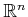. Assume that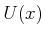and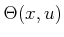are finite. The value-iteration methods of Section 10.2.1 can be directly applied by using the interpolation concepts from Section 8.5.2 to compute the cost-to-go values over. In the nondeterministic case, the recurrence is (10.39), in which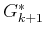is represented on a finite sample set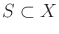and is evaluated on all other points in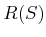by interpolation (recall from Section 8.5.2 thatis the interpolation region of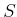). In the probabilistic case, (10.45) or (10.46) may once again be used, butis evaluated by interpolation.

Ifis continuous, then it can be sampled to evaluate the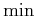in each recurrence, as suggested in Section 8.5.2. Now supposeis continuous. In the nondeterministic case,can be sampled to evaluate the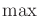in (10.39) or it may be possible to employ a general optimization technique directly over. In the probabilistic case, the expectation must be taken over a continuous probability space. A probability density function,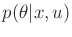, characterizes nature's action. A probabilistic state transition density function can be derived from this as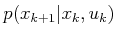. Using these densities, the continuous versions of (10.45) and (10.46) become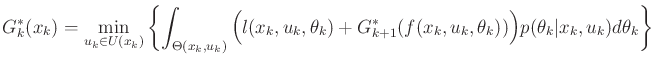(10.121)

and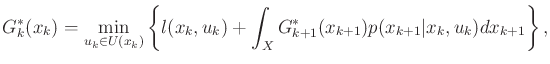(10.122)

respectively. Sampling can be used to evaluate the integrals. One straightforward method is to approximateby a discrete distribution. For example, in one dimension, this can be achieved by partitioninginto intervals, in which each interval is declared to be a discrete nature action. The probability associated with the discrete nature action is just the integral ofover the associated interval.

Section 8.5.2 concluded by describing Dijkstra-like algorithms for continuous spaces. These were derived mainly by using backprojections, (8.66), to conclude that some samples cannot change their values because they are too far from the active set. The same principle can be applied in the current setting; however, the weak backprojection, (10.20), must be used instead. Using the weak backprojection, the usual value iterations can be applied while removing all samples that are not in the active set. For many problems, however, the size of the active set may quickly become unmanageable because the weak backprojection often causes much faster propagation than the original backprojection. Continuous-state generalizations of the Dijkstra-like algorithms in Section 10.2.3 can be made; however, this requires the additional condition that in every iteration, it must be possible to extend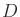by forcing the next state to lie in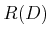, in spite of nature.

Steven M LaValle 2020-08-14